# Part 3.6The Golden Ratio

## Calculating the Golden Ratio

The Golden Ratio is defined as below:

$$φ = {a + b \over a} = 1.61803398875...$$

Where a is the large section and b is the smaller.

This 1.618... number is known as the Golden Number or the Greek letter φ (Phi).

By rearranging the mathematical formula before it is possible to get the value of b:

$$b = {a \over φ} - a$$

Using this formula, it is possible to get the value of b when a is 700:

$${700 \over φ} - 700 = 432.6237923$$

To ensure this is correct, put the values into the original formula again:

$${700 + 432.6237923 \over 700} = φ$$

An alternative way to this is to the Fibonacci sequence of numbers in pairs:

The first ten Fibonnaci numbers are defined as follows:

1, 2, 3, 5, 8, 13, 21, 34, 55, 89

Ignoring the first two and beginning with the pair (3, 5) it can be shown that this obeys the Golden Ratio since 5 / 3 is ~1.6. This can be proven with the pair (8, 13) since 13 / 8 is ~1.6. In fact, any one of these pairs of numbers can be used since they all obey the Golden Ratio.

Fibonacci numbers are calculated as follows:

$$f(n) = f(n-1) + f(n-2)$$

Where f is a mathematical function that returns a Fibonacci number at a position n.

What this means is that each Fibonacci number is the sum of the previous two numbers. For example:

89 = 55 + 34
13 = 8 + 5

However, Fibonacci numbers can also be calculated using φ:

89 = 55 * φ
13 = 8 * φ

All values should be rounded down to get an accurate result.

The Golden Ratio is one of the most fundamental concepts used in design.

The Golden Ratio creates boxes of a certain size. The first and larger box is called a and the second smaller box is called b.

Following the Fibonacci sequence and using the Golden Number, a box model as below can be generated: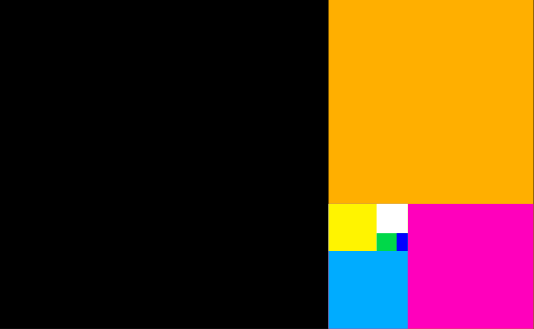The Golden Ratio used to create a box model

This box model is formed by creating a large object (box a) then creating a smaller box (box b) which takes up approximately 39% of this box. It is repeated with the smaller box becoming box a and the smaller box being rotated 90 degrees from the previous box. This process is then repeated constantly:

Stage 1

Box a is placed.Stage 2

Now place the smaller box b with a width of the black box divided by φ.Stage 3

Now inside the orange box, place a new box and make it's height the height of the orange box divided by φ at the bottom of the box (this is now a rotated rectangle).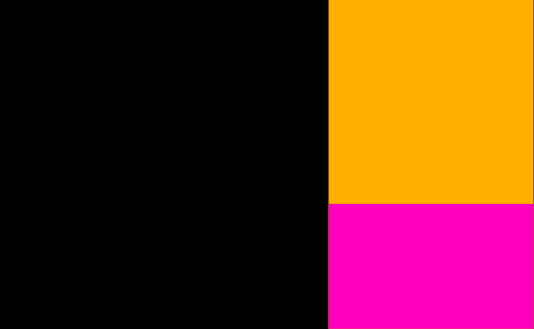Keep repeating the process described before (remembering to rotate after each stage):

Stage 4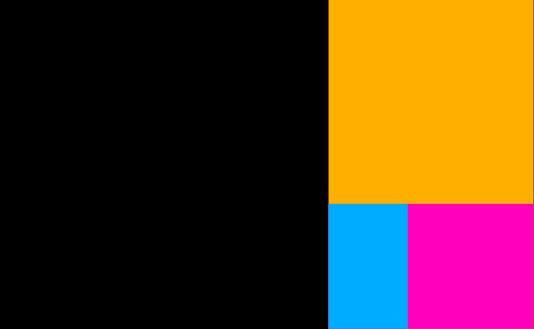Stage 5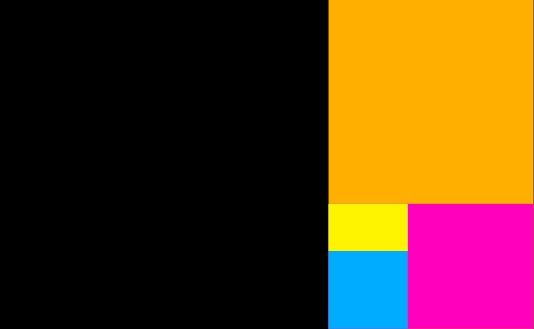Stage 6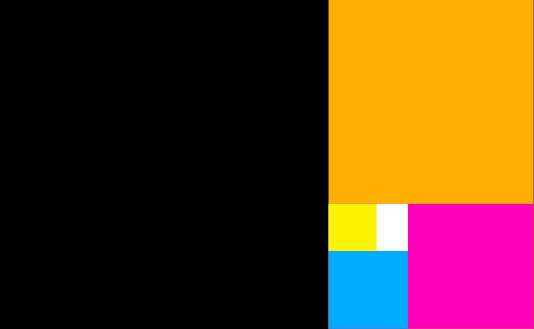Stage 7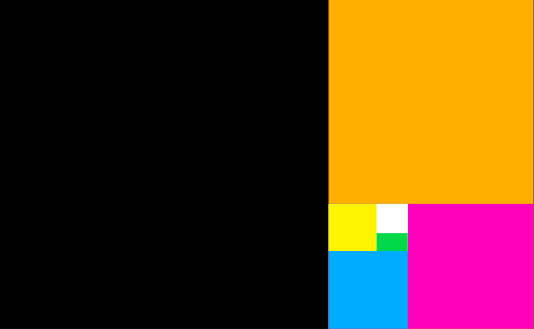Stage 8## The Golden Ratio in nature

The ratio is found across nature in many different places and one such place is in nature. Take for instance, a snail:A snail's shell shows the Golden Ratio in nature.

Observe how this fits into the box model shown previously:The Golden Ratio shows how a spiral design fits perfectly into the model

## The Golden Ratio in web design

Many websites implement the Golden Ratio. It's an important design consideration and whilst this website does not (it has a fixed sidebar and therefore cannot) it did originally (but since the sidebar has fixed width content there was no need).

In relation to web design, a sidebar could be formed using the Golden Ratio: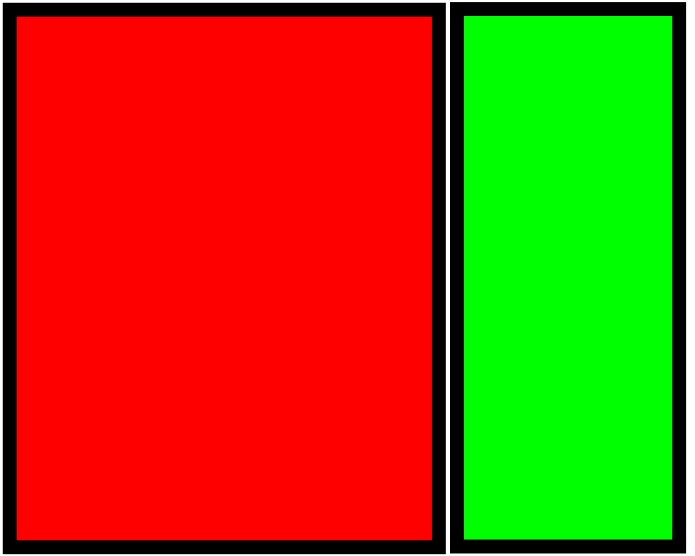The layout of a website with a sidebar and main contents section following the Golden Ratio

This website employs the Golden Ratio by making the contents 1.62 times the size of the sidebar. Assuming the sidebar was 300 pixels in width, the content section would 486 pixels in width. This is how the Golden Ratio can be applied to web design.

This equates roughly to 61% dedicated to the main content and 39% to the sidebar.

Assuming a webpage has a content of 712 pixels, calculating the appropriate size of the sidebar would be done by using the following formula:

$$sidebar = {712\,pixels \over φ} = 439\,pixels$$

This rule is not limited to a sidebar. In fact, the Golden Ratio can be applied anywhere on a website.

## Why bother with the Golden Ratio?

The Golden Ratio is everywhere in nature and mathematics and now website design. It plays a huge role in web design just as it does in the other disciples it appears in because of a very important way in which users think.

The Golden Ratio on a website encourages users to look to the larger section but to know naturally that the sidebar (or side content) exists as additional, less important information. It encourages users to see the main event before seeing the contextual information that may exist in the smaller section. This is important from a marketing perspective.

The Golden Ratio is just one way of making parts of a website look more important than others.

Code preview
Feedback 👍
Comments are sent via email to me.